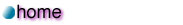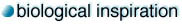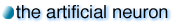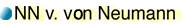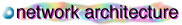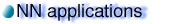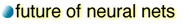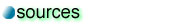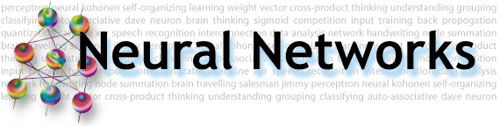Some specific details of neural networks:

Although the possibilities of solving problems using a single perceptron is limited, by arranging many perceptrons in various configurations and applying training mechanisms, one can actually perform tasks that are hard to implement using conventional Von Neumann machines.

We are going to describe four different uses of neural networks that are of great significance:

1. Classification. In a mathematical sense, this involves dividing an n-dimensional space into various regions, and given a point in the space one should tell which region to which it belongs. This idea is used in many real-world applications, for instance, in various pattern recognition programs. Each pattern is transformed into a multi-dimensional point, and is classified to a certain group, each of which represents a known pattern.

 Type of network used: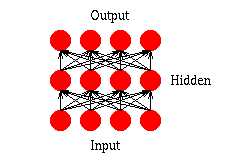Feed-forward networks

2. Prediction. A neural network can be trained to produce outputs that are expected given a particular input. If we have a network that fits well in modeling a known sequence of values, one can use it to predict future results. An obvious example is stock market prediction.

 Type of network used:Feed-forward networks

3. Clustering. Sometimes we have to analyze data that are so complicated there is no obvious way to classify them into different categories. Neural netowrks can be used to identify special features of these data and classify them into different categories without prior knowledge of the data. This technique is useful in data-mining for both commercial and scientific uses.

 Type of network used: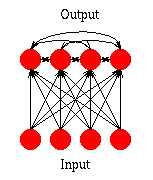Simple Competitive NetworksAdaptive Resonance Theory (ART) networks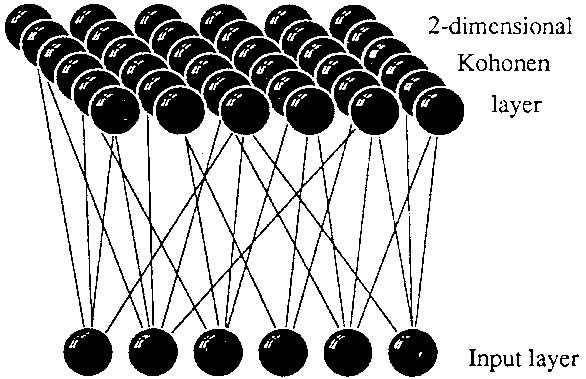Kohonen Self-Organizing Maps (SOM)

4. Association. A neural network can be trained to "remember" a number of patterns, so that when a distorted version of a particular pattern is presented, the network associates it with the closest one in its memory and returns the original version of that particular pattern. This is useful for restoring noisy data.

 Type of network used: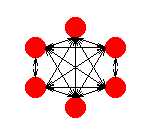Hopfield networks

The above is just a general picture of what neural networks can do in real life. There are many creative uses of neural networks that arises from these general applications. One example is image compression using association networks; another is solving the Travelling Salesman's Problem using clustering networks.